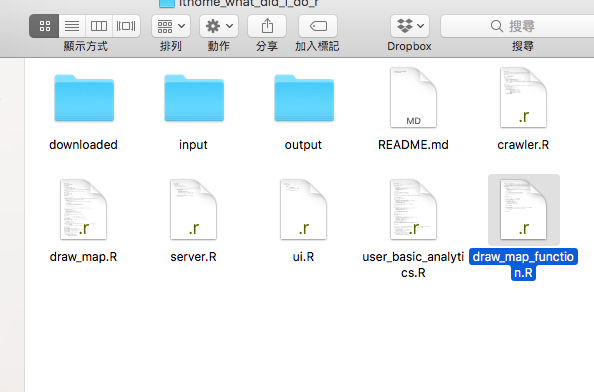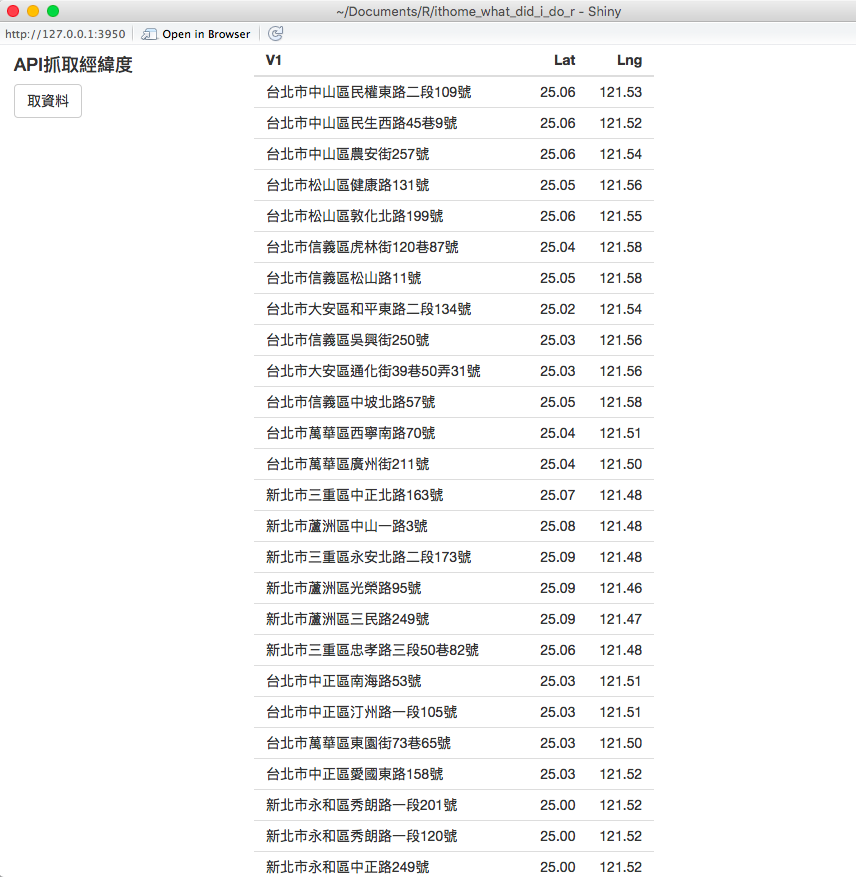#2018 iT 邦幫忙鐵人賽DAY 25
3
Data Technology

## 【25】當老闆扎了兩下眼問：那我們手邊資料有什麼情境適合這樣分段處理的嗎？

``````library(shiny)
shinyUI(fluidPage(
fluidRow(
column(3,
h4("API抓取經緯度"),
actionButton("request", "取資料")
),
column(9,
tableOutput('addressTable')
)
)
))
````````````library(shiny)
library(tidyr)
library(dplyr)
source("draw_map_function.R")
shinyServer(function(input, output) {

v <- reactiveValues(address = read.csv("input/address.csv", stringsAsFactors=FALSE, header=FALSE))

})
``````

``````observeEvent(input\$request, {
v\$addressWithLatLng <- v\$address %>%
rowwise() %>%
mutate(LatLng = getLatLng(V1)) %>%
filter(LatLng!="error") %>%
separate(LatLng, c("Lat", "Lng"), sep=",") %>%
mutate(Lat=as.numeric(Lat), Lng=as.numeric(Lng))
})
``````

``````output\$addressTable <- renderTable({
if (is.null(v\$addressWithLatLng)) return(v\$address)
v\$addressWithLatLng
})
``````

1.一開始的地址資料。2.按下取資料後的狀態。ref:
day25原始碼